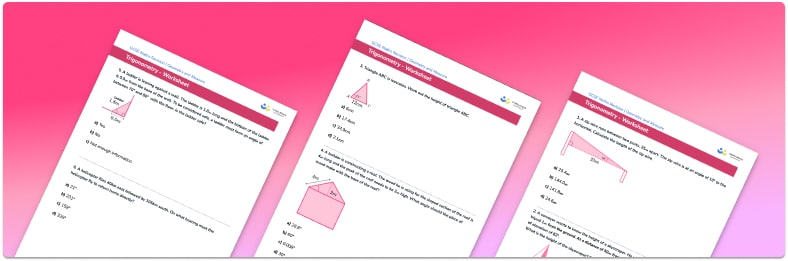# Trigonometry Worksheet• Section 1 of the trigonometry worksheet contains 36 skills-based trigonometry questions, in 3 groups to support differentiation
• Section 2 contains 4 applied trigonometry questions with a mix of worded problems and deeper problem solving questions
• Section 3 contains 4 foundation and higher level GCSE exam style trigonometry questions
• Answers and a mark scheme for all trigonometry questions are provided
• Questions follow variation theory with plenty of opportunities for students to work independently at their own level
• All questions created by fully qualified expert secondary maths teachers
• Suitable for GCSE maths revision for AQA, OCR and Edexcel exam boards

• This field is for validation purposes and should be left unchanged.

You can unsubscribe at any time (each email we send will contain an easy way to unsubscribe). To find out more about how we use your data, see our privacy policy.

### Trigonometry at a glance

Trigonometry is the relationship between the sides and angles in  triangles. There are three trigonometric ratios we need to be aware of at GCSE: sine, cosine and tangent (abbreviated sin, cos, tan). Each trig ratio gives the relationship between an angle and two particular sides of a right angled triangle – for example, sine involves the ratio of the opposite side and the hypotenuse. The mnemonic SOHCAHTOA is used to remember which trig ratio is used for which pair of sides.

Simple trigonometry (SOHCAHTOA) can be used to find the length of a missing side in a right angle triangle. By using the inverse trig operation (notation sin-1, cos-1, tan-1), we can also find the size of a missing angle.

With the exception of a few angles, trigonometric functions often to return non-terminating decimals. It is therefore important that students are confident with rounding their answers to a suitable number of decimal places.

There are three more advanced rules using trigonometry at GCSE – these are the sine rule (law of sines), the cosine rule (law of cosines), and the trigonometric area formula for area of a triangle. These rules apply to any triangle, not just right angled triangles. The cosine rule, for example, can be used to find the length of a missing side, when two side lengths and the size of the angle between them is known.

While students do not need to know trigonometric identities at GCSE, knowledge of the identity tan(x) is equivalent to sin(x) divided by cos(x) can be useful for remembering some exact trig values.

Looking forward, students can then progress to additional geometry worksheets, for example a 3D Pythagoras worksheet,For more teaching and learning support on Geometry our GCSE maths lessons provide step by step support for all GCSE maths concepts.

## Do you have KS4 students who need more focused attention to succeed at GCSE?There will be students in your class who require individual attention to help them succeed in their maths GCSEs. In a class of 30, it’s not always easy to provide.

Help your students feel confident with exam-style questions and the strategies they’ll need to answer them correctly with our dedicated GCSE maths revision programme.

Lessons are selected to provide support where each student needs it most, and specially-trained GCSE maths tutors adapt the pitch and pace of each lesson. This ensures a personalised revision programme that raises grades and boosts confidence.

Find out more